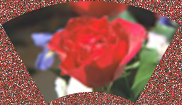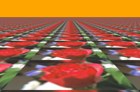# Distortion¶

ImageMagick provides several ways to distort an image by applying various transformations against user-supplied arguments. In Wand, the method Image.distort is used, and follows a basic function signature of:

with Image(...) as img:
img.distort(method, arguments)


Where method is a string provided by DISTORTION_METHODS, and arguments is a list of doubles. Each method parses the arguments list differently. For example:

# Arc can have a minimum of 1 argument
img.distort('arc', (degree, ))
# Affine 3-point will require 6 arguments
points = (x1, y1, x2, y2,
x3, y3, x4, y4,
x5, y5, x6, y6)
img.distort('affine', points)


A more complete & detailed overview on distortion can be found in Distorting Images usage article by Anthony Thyssen.

## Controlling Resulting Images¶

### Virtual Pixels¶

When performing distortion on raster images, the resulting image often includes pixels that are outside original bounding raster. These regions are referred to as vertical pixels, and can be controlled by setting Image.virtual_pixel to any value defined in VIRTUAL_PIXEL_METHOD.

Virtual pixels set to 'transparent', 'black', or 'white' are the most common, but many users prefer use the existing background color.

with Image(filename='rose:') as img:
img.resize(140, 92)
img.background_color = img[70, 46]
img.virtual_pixel = 'background'
img.distort('arc', (60, ))Other virtual_pixel values can create special effects.

 Virtual Pixel Example ditheredgemirrorrandomtile### Matte Color¶

Some distortion transitions can not be calculated in the virtual-pixel space. Either being invalid, or NaN (not-a-number). You can define how such a pixel should be represented by setting the Image.matte_color property.

from wand.color import Color
from wand.image import Image

with Image(filename='rose:') as img:
img.resize(140, 92)
img.matte_color = Color('ORANGE')
img.virtual_pixel = 'tile'
args = (0, 0, 30, 60, 140, 0, 110, 60,
0, 92, 2, 90, 140, 92, 138, 90)
img.distort('perspective', args)### Rendering Size¶

Setting the 'distort:viewport' artifact allows you to define the size, and offset of the resulting image:

img.artifacts['distort:viewport'] = '300x200+50+50'


Setting the 'distort:scale' artifact will resizing the final image:

img.artifacts['distort:scale'] = '75%'


## Scale Rotate Translate¶

A more common form of distortion, the method 'scale_rotate_translate' can be controlled by the total number of arguments.

The total arguments dictate the following order.

 Total Arguments Argument Order 1 Angle 2 Scale, Angle 3 X, Y, Angle 4 X, Y, Scale, Angle 5 X, Y, ScaleX, ScaleY, Angle 6 X, Y, Scale, Angle, NewX, NewY 7 X, Y, ScaleX, ScaleY, Angle, NewX, NewY

For example…

A single argument would be treated as an angle:

from wand.color import Color
from wand.image import Image

with Image(filename='rose:') as img:
img.resize(140, 92)
img.background_color = Color('skyblue')
img.virtual_pixel = 'background'
angle = 90.0
img.distort('scale_rotate_translate', (angle,))Two arguments would be treated as a scale & angle:

with Image(filename='rose:') as img:
img.resize(140, 92)
img.background_color = Color('skyblue')
img.virtual_pixel = 'background'
angle = 90.0
scale = 0.5
img.distort('scale_rotate_translate', (scale, angle,))And three arguments would describe the origin of rotation:

with Image(filename='rose:') as img:
img.resize(140, 92)
img.background_color = Color('skyblue')
img.virtual_pixel = 'background'
x = 80
y = 60
angle = 90.0
img.distort('scale_rotate_translate', (x, y, angle,))… and so forth.

## Perspective¶

Perspective distortion requires 4 pairs of points which is a total of 16 doubles. The order of the arguments are groups of source & destination coordinate pairs.

src1x, src1y, dst1x, dst1y,
src2x, src2y, dst2x, dst2y,
src3x, src3y, dst3x, dst3y,
src4x, src4y, dst4x, dst4y


For example:

from itertools import chain
from wand.color import Color
from wand.image import Image

with Image(filename='rose:') as img:
img.resize(140, 92)
img.background_color = Color('skyblue')
img.virtual_pixel = 'background'
source_points = (
(0, 0),
(140, 0),
(0, 92),
(140, 92)
)
destination_points = (
(14, 4.6),
(126.9, 9.2),
(0, 92),
(140, 92)
)
order = chain.from_iterable(zip(source_points, destination_points))
arguments = list(chain.from_iterable(order))
img.distort('perspective', arguments)## Affine¶

Affine distortion performs a shear operation. The arguments are similar to perspective, but only need a pair of 3 points, or 12 real numbers.

src1x, src1y, dst1x, dst1y,
src2x, src2y, dst2x, dst2y,
src3x, src3y, dst3x, dst3y


For example:

from wand.color import Color
from wand.image import Image

with Image(filename='rose:') as img:
img.resize(140, 92)
img.background_color = Color('skyblue')
img.virtual_pixel = 'background'
args = (
10, 10, 15, 15,  # Point 1: (10, 10) => (15,  15)
139, 0, 100, 20, # Point 2: (139, 0) => (100, 20)
0, 92, 50, 80    # Point 3: (0,  92) => (50,  80)
)
img.distort('affine', args)## Affine Projection¶

Affine projection is identical to Scale Rotate Translate, but requires exactly 6 real numbers for the distortion arguments.

Scalex, Rotatex, Rotatey, Scaley, Translatex, Translatey


For example:

from collections import namedtuple
from wand.color import Color
from wand.image import Image

Point = namedtuple('Point', ['x', 'y'])

with Image(filename='rose:') as img:
img.resize(140, 92)
img.background_color = Color('skyblue')
img.virtual_pixel = 'background'
rotate = Point(0.1, 0)
scale = Point(0.7, 0.6)
translate = Point(5, 5)
args = (
scale.x, rotate.x, rotate.y,
scale.y, translate.x, translate.y
)
img.distort('affine_projection', args)